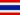4.3 Realizar tareas matemáticas# 4.3 Realizar tareas matemáticas

• Study Reminders

We'll email you at these times to remind you to study

You can set up to 7 reminders per week

#### You're all set

We'll email you at these times to remind you to study

Monday

Tuesday

Wednesday

Thursday

Friday

Saturday

Sunday

• Apuntes
• Revisión por temaWAI P. 0 0 Lesson 4 - Introduction to Spreadsheet Programs 4.3 - Performing Mathematical Tasks Performing Mathematical Tasks An important advantage of a spreadsheet is that apart from storing and manipulating data, you can also use it to perform various mathematical tasks on the stored data quickly and accurately. To perform these mathematical tasks, you use functions provided by the spreadsheet program. A function is an operation that is performed on the data in the cells by using calculation tools available in the program. For example, after recording your household expenses in a spreadsheet, you can use the AVERAGE function to calculate the average amount you spent for groceries. You can also create your own formulas to perform mathematical calculations. Using formulas and functions in a spreadsheet helps you to easily modify any data in your calculations. This is because formulas and functions refer to the cell address, not the data in the cells. When you change the data in a cell, the applied formula or the function does not change, and the result of the formula is updated automatically. In this simulated lab, you will perform basic mathematical tasks in an Excel 2007 spreadsheet. Click to launch the lab. Follow the steps to complete the lab.WAI P. 1 0 Describe tbe lession 4?
• Text Version